# WBJEE 2017 Physics Paper with Solutions

WBJEE 2017 Physics paper solutions are given on this page. BYJU’S provides step by step solutions so that students can understand the problems clearly. Students are advised to learn these solutions to score higher ranks for the upcoming WBJEE exams. Previous year papers are an important aid for the students to increase speed and accuracy and thus improve their confidence. This helps students to be stress-free during the exam. They can easily download the PDF format for free.

### WBJEE 2017 - Physics

Question 1: The velocity of a particle executing a simple harmonic motion is 13 ms–1, when its distance from the equilibrium position (Q) is 3 m and its velocity is 12 ms–1, when it is 5 m away from Q. The frequency of the simple harmonic motion is

1. a. 5 π/8
2. b. 5/8 π
3. c. 8 π/5
4. d. 8/5 π

Solution:

Velocity of particle at any x from mean-position executing SHM is:-

v = ω√(A2-x2)

(v2/ ω2)+x2 = A2

Given:

At x = 3 m; v = 13 ms-1

(132/ ω2)+32 = A2…(1)

At x = 5 m; v = 12 ms-1

(122/ ω2)+52 = A2…(2)

From (1) and (2)

(169/ ω2)+9 = (144/ ω2)+25

(169/ ω2)- (144/ ω2) = 25-9

(25)/ ω2 = 16

ω2 = 25/16

ω = (5/4) rad/sec

Frequency [f]:

ω = 2πf

f = ω/2π

= 5/8π

Question 2: A uniform string of length L and mass M is fixed at both ends while it is subject to a tension T. It can vibrate at frequencies (ν) given by the formula (where n = 1, 2, 3, .....)

1. a. ν = (n/2) √(T/ML)
2. b. ν = (n/2L) √(T/M)
3. c. ν = (1/2n) √(T/ML)
4. d. ν = (n/2) √(TL/M)

Solution:

If the string is vibrating in n segment and wavelength of wave is λ.

L = nλ/2

Velocity of transverse wave in string is:-

v = √T/ μ

T = Tension in the string

μ = mass per unit length = M/L

Velocity of a wave:-

V = νλ

V = v/λ

= (n/2L)√(TL/M)

= (n/2)(√T/ML)

Question 3: A uniform capillary tube of length l and inner radius r with its upper end sealed is submerged vertically into water. The outside pressure is p0 and surface tension of water is γ. When a length x of the capillary is submerged into water, it is found that water levels inside and outside the capillary coincide. The value of x is

1. a. l/(1+p0r/4 γ)
2. b. l(1- p0r/4 γ )
3. c. l(1- p0r/2γ)
4. d. l/(1+p0r/2γ)

Solution: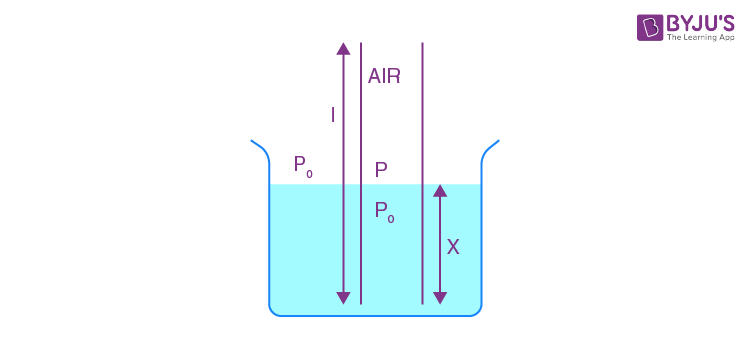Assumption:-

The temperature of Air in the capillary remains constant

[PV = nRT]

So for air inside capillary

PiVi = PfVf

P0Al = PA[ l– x]

P = P0l/(l-x)…..(i)

[Angle of contact θ = 0°]

At interface:- P-P0 = 2γ/r…..(ii)

Put value of P from (i) in equation (ii):-

(P0l/(l-x))-P0 = 2γ/r

(P0x/(l-x)) = 2γ/r

P0r/2 γ = (l-x)/x

P0r/2 γ = (l/x)-1

(P0r/2 γ)+1 = (l/x)

x = l/(1+P0r/2 γ)

Question 4: A liquid of bulk modulus k is compressed by applying an external pressure such that its density increases by 0.01%. The pressure applied on the liquid is

1. a. k/10000
2. b. k/1000
3. c. 1000k
4. d. 0.01k

Solution:

Bulk modulus [K] = -P/ ∆V/V …..(i)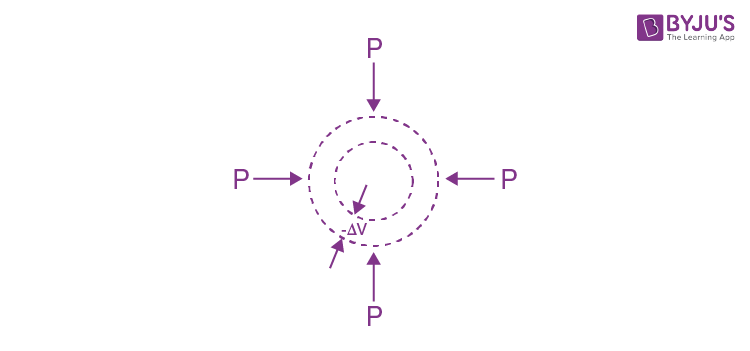Mass of liquid element is constant m = ρV

∆m/m = ∆ρ/ρ+∆V/V

0 = ∆ρ/ρ+∆V/V

∆ρ/ρ = -∆V/V

From (i) P = -K∆V/V

P = +K∆ρ/ρ

∆ρ/ρ = 0.01/100 = 1/10000

P = K/10000

Question 5: Temperature of an ideal gas, initially at 270C, is raised by 60C. The rms velocity of the gas molecules will,

1. a. increase by nearly 2%
2. b. decrease by nearly 2%
3. c. increase by nearly 1%
4. d. decrease by nearly 1%

Solution:

The rms velocity of an ideal gas:

vrms = √(3RT/M)

vrms ∝ √T

For % change in rms velocity

∆v/v = (½)∆T/T

= ½ (6/300)

= 1/100

%∆v/v = 1%

Question 6: 2 moles of an ideal monoatomic gas is carried from a state (P0, V0) to a state (2P0, 2V0) along a straight line path in a P-V diagram. The amount of heat absorbed by the gas in the process is given by

1. a. 3P0V0
2. b. (9/2) P0V0
3. c. 6 P0V0
4. d. (3/2)P0V0

Solution: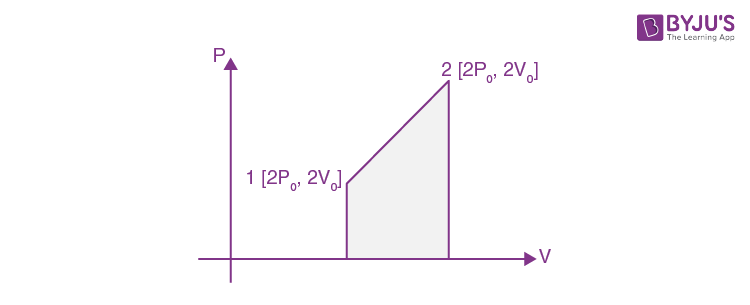Given:-

n = 2 moles

[Ideal monoatomic gas] , Cv = 3R/2

Work done by the gas = Area enclosed by curve on volume axis

W = (½)[P0+2P0].v0

= 3P0V0/2

∆U = nCv ∆T

= n(3R/2)[T2-T1]

= n(3R/2)[(2P02V0/nR)-( P0V0/nR)]

= 9P0V0

For a process:

∆Q = W+∆U

∆Q = (3P0V0)/2 +(9P0V0)/2

= 6P0V0

Question 7: A solid rectangular sheet has two different coefficients of linear expansion α1 and α2 along its length and breadth respectively. The coefficient of surface expansion is (for α1t <<1, α2 t < < 1)

1. a. (α1+ α2)/2
2. b. 2(α1+ α2)
3. c. 4 α1α2/(α1+ α2)
4. d. (α1+ α2)

Solution: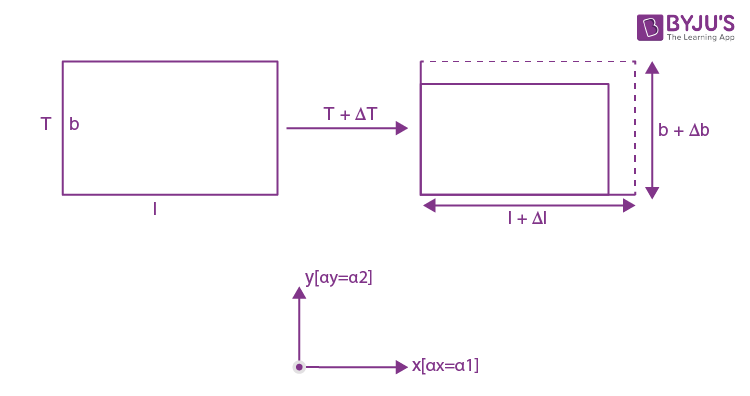A = lb

∆ A/A = (∆l/l)+( ∆b/b)

l = l α1 ∆T

∆b = b α2 ∆T

∆ A/A = α1 ∆T+ α2 ∆T …(i)

If β is Arial co-efficient of solid then,

∆A = βA ∆T

β∆T = α1 ∆T+ α2 ∆T ..(ii)

From (i) and (ii)

β = α1+ α2

Question 8: A positive charge Q is situated at the centre of a cube. The electric flux through any face of the cube is (in SI units)

1. a. Q/6ε0
2. b. 4 πQ
3. c. Q/4πε0
4. d. Q/6πε0

Solution: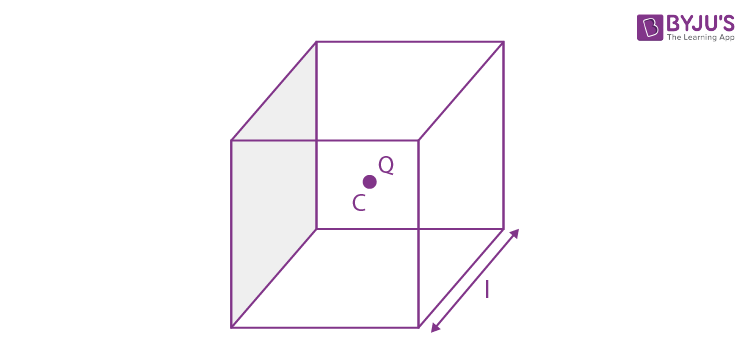From Gauss law electric flux through any closed surface is given by:-

φ = qin/ ε0

φ = Q/ ε0

As charge is at the body centre of the cube hence, flux passing through each face is same according to symmetricity.

φface = Q/ 6ε0

Question 9: Three capacitors of capacitance 1.0, 2.0 and 5.0 µF are connected in series to a 10V source. The potential difference across the 2.0 µF capacitor is

1. a. (100/17)V
2. b. (20/17)V
3. c. (50/17)V
4. d. 10V

Solution: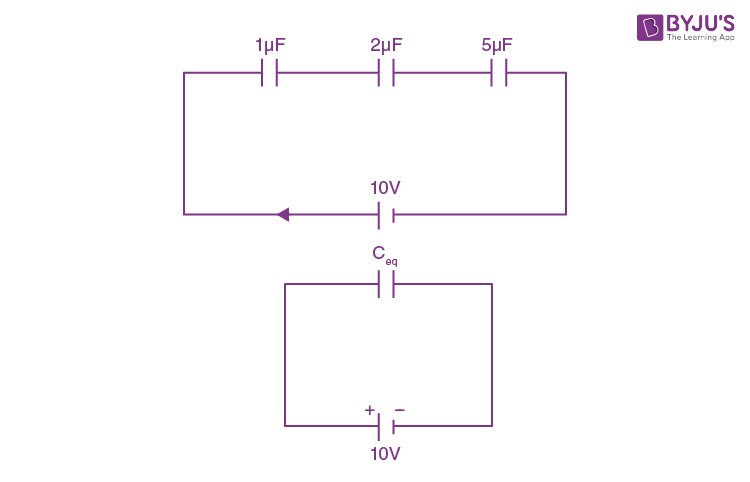1/Ceq = (1/C1)+(1/C2)+(1/C3)

1/Ceq = (1/1)+(1/2)+(1/5)

1/Ceq = 1+(1/2)+(1/5)

Ceq = 10/17 μF

Charge – flown by battery:-

Q = CeqV

= (10/17)10

= 100/17 μC

As all capacitors are connected in series charge is same across all capacitors.

For 2 μF

q2 = Q = C2V2

V2 = Q/C2

= (100/17)/2

= 50/17 volt

Question 10: A charge of 0.8 coulomb is divided into two charges Q1 and Q2. These are kept at a separation of 30 cm. The force on Q1 is maximum when

1. a. Q1 = Q2 = 0.4C
2. b. Q1 ≈ 0.8C, Q2 negligible
3. c. Q1 negligible, Q2 ≈ 0.8C
4. d. Q1 = 0.2 C, Q2 = 0.6 C

Solution:Q = Q1+Q2

Q2 = Q-Q1

Force on charge Q1

F = KQ1Q2/r2

F = KQ1[Q-Q1]/r2

For Fmax

dF/dQ1 = 0

Q-2Q1 = 0

Q1 = Q/2 = 0.4 C

Question 11: The magnetic field due to a current in a straight wire segment of length L at a point on its perpendicular bisector at a distance r (r >> L)

1. a. decreases as 1/r
2. b. decreases as 1/r2
3. c. decreases as 1/r3
4. d. approaches a finite limit as r tends to infinity.

Solution: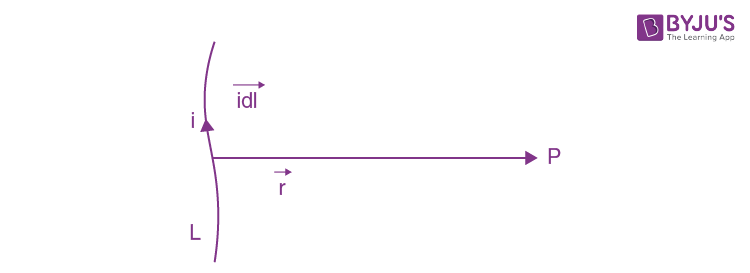By Biot - Savart law:-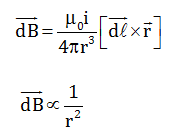Question 12: The magnets of two suspended coil galvanometers are of the same strength so that they produce identical uniform magnetic fields in the region of the coils. The coil of the first one is in the shape of a square of side a and that of the second one is circular of radius a/ √π . When the same current is passed through the coils, the ratio of the torque experienced by the first coil to that experienced by the second one is

1. a. 1 :1/√π
2. b. 1 : 1
3. c. π : 1
4. d. 1 : π

Solution:

Torque [τ] = iAB

As i and B are same

So τ ∝ A

τ12 = A1/A2

τ12 = a2/( π(a/√π)2

= 1

Question 13: A proton is moving with a uniform velocity of 106 ms–1 along the Y-axis, under the joint action of a magnetic field along Z-axis and an electric field of magnitude 2 × 104 Vm–1 along the negative X-axis. If the electric field is switched off, the proton starts moving in a circle. The radius of the circle is nearly (given : ratio for proton = 108 Ckg–1)

1. a. 0.5 m
2. b. 0.2 m
3. c. 0.1m
4. d. 0.05 m

Solution:

We know from EM waves:

$$\left | \vec{E} \right |= \left | \vec{B} \right |V$$

B = E/V = (2×104)/106

B = 2×10-2 T

Now when E is switched off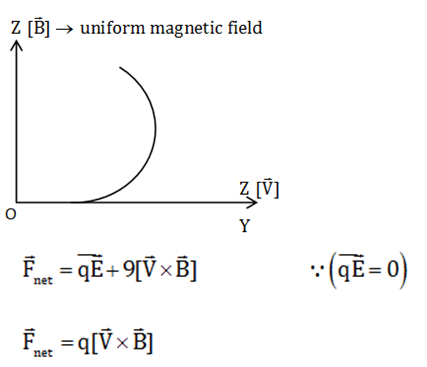For circular motion

mv2/R = qvB

Given m/a = 10-8Ckg-1

R = mv/qB

= 10-8×106/2×10-2

= 0.5 m

Question 14: When the frequency of the AC voltage applied to a series LCR circuit is gradually increased from a low value, the impedance of the circuit

1. a. monotonically increases
2. b. first increases and then decreases
3. c. first decreases and then increases
4. d. monotonically decreases

Solution:

The impedance of the circuit [series LCR] is given by:-

Z2 = [XL-XC]2+R2

Z2 = [ωL- 1/ωC]2+R2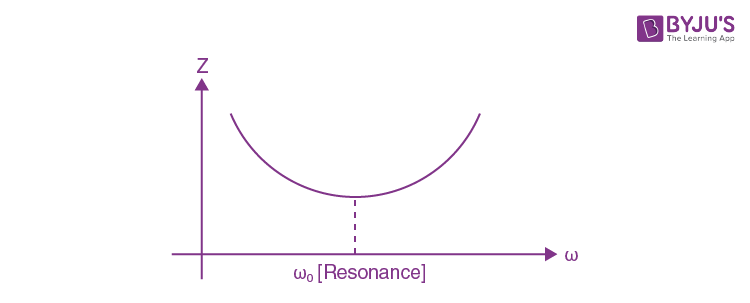As we gradually increase frequency, z first decreases and then increases.

Question 15: Six wires, each of resistance r, are connected so as to form a tetrahedron. The equivalent resistance of the combination when current enters through one corner and leaves through some other corner is

1. a. r
2. b. 2r
3. c. r/3
4. d. r/2

Solution: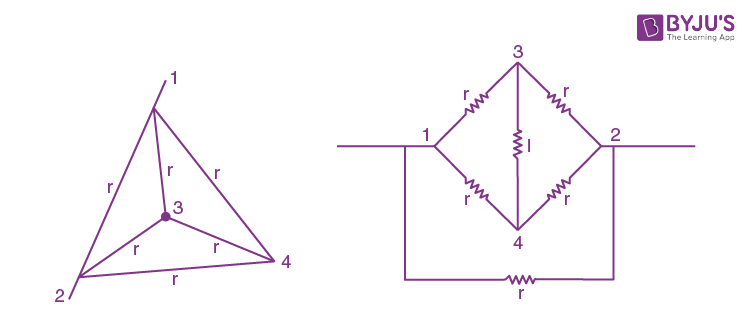3 & 4 are equipotential point then,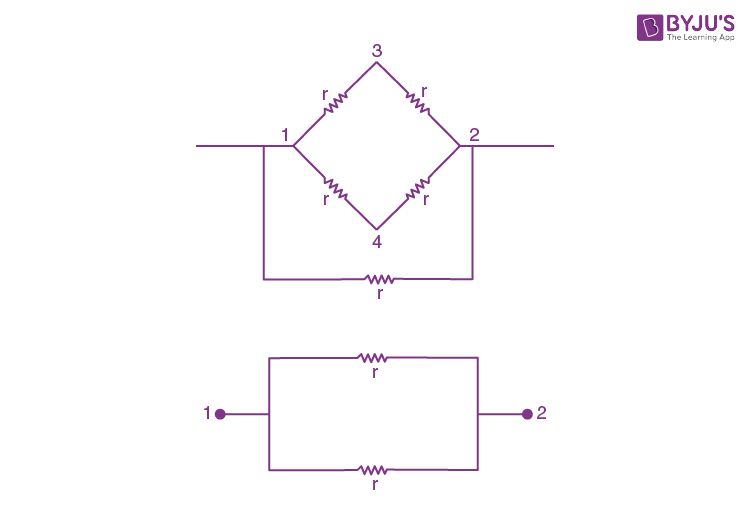Req = r/2

Question 16: A Consider the circuit shown in the figure. The value of the resistance X for which the thermal power generated in it is practically independent of small variation of its resistance is1. a. X = R
2. b. X = R/3
3. c. X = R/2
4. d. X = 2R

Solution: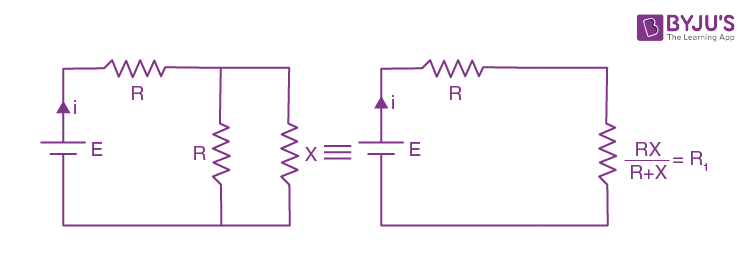i = E/[R+(RX/R+X)]

Voltage drop across [R1]:

VR1 = iR1

= E/[R+(RX/R+X)](RX/R+X)

VR1 = EX/(R+2X)

PX = VR12/X

PX = E2X/(R+2X)2

dPX/dx = E2[R-2X]/[R+2X]3

[dPX] will be zero for all dx if

X = R/2

Question 17: Consider the circuit shown in the figure where all the resistances are of magnitude 1kilo ohm. If the current in the extreme right resistance X is 1 mA, the potential difference between A and B is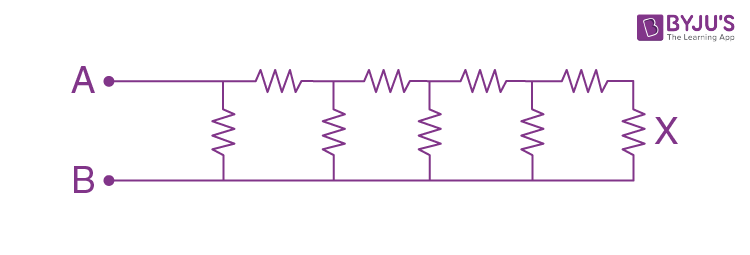1. a. 34 V
2. b. 21 V
3. c. 38 V
4. d. 55 V

Solution: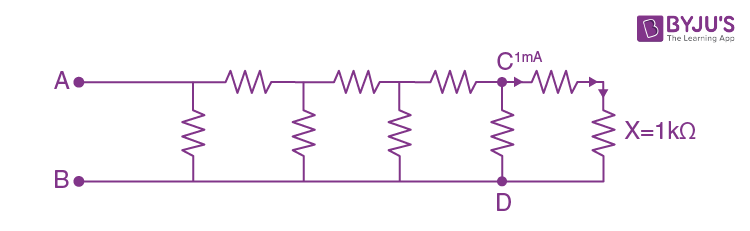Across C & D same potential current will divide according to :-

i ∝1/R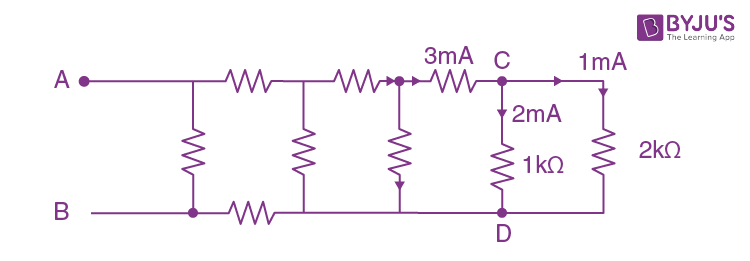At C apply KCL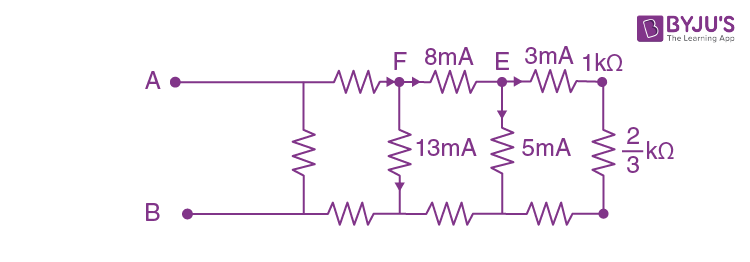Apply KCL at E.

Apply KCl at F.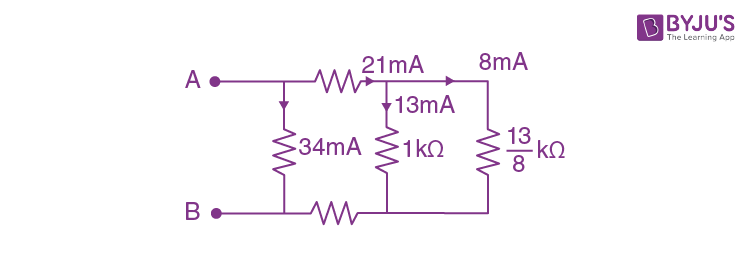VAB = iR = 34×10–3A×1×103 ohm

= 34 V

Question 18: The ratio of the diameter of the sun to the distance between the earth and the sun is approximately 0.009. The approximate diameter of the image of the sun formed by a concave spherical mirror of radius of curvature 0.4 m is

1. a. 4.5 × 10–6 m
2. b. 4.0 × 10–6 m
3. c. 3.6 × 10–3 m
4. d. 1.8 × 10–3 m

Solution: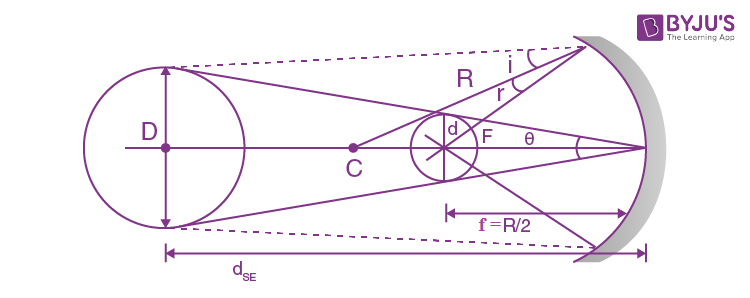For concave-mirror if object is placed at infinity: image will be formed at focus [f]

θ = D/dSE = d/f

d = θf

d = 0.009×0.2 m

d = 1.8×10–3 m

Question 19: Two monochromatic coherent light beams A and B have intensities L and L/4 respectively. If these beams are superposed, the maximum and minimum intensities will be

1. a. 9L/4, L/4
2. b. 5L/4, 0
3. c. 5L/2, 0
4. d. 2L,L/2

Solution:

I = √I12+√I22+2√I1√I2 cos φ

For max. intensity

cos φ = 1

Imax = (√I1 + √I2)2

For minimum intensity:

Imin = (√I1 - √I2)2

Imax = (√L + √(L/4))2

= 9L/4

Imin = (√L - √(L/4))2

= L/4

Question 20: A point object is held above a thin equiconvex lens at its focus. The focal length is 0.1 m and the lens rests on a horizontal thin plane mirror. The final image will be formed at

1. a. infinite distance above the lens
2. b. 0.1 m above the center of the lens
3. c. infinite distance below the lens
4. d. 0.1 m below the center of the lens

Solution:Image will be formed on object itself.

Question 21: A parallel beam of light is incident on a glass prism in the shape of a quarter cylinder of radius R = 0.05 m and refractive index n = 1.5 placed on a horizontal table as shown in the figure. Beyond the cylinder, a patch of light is found whose nearest distance x from the cylinder is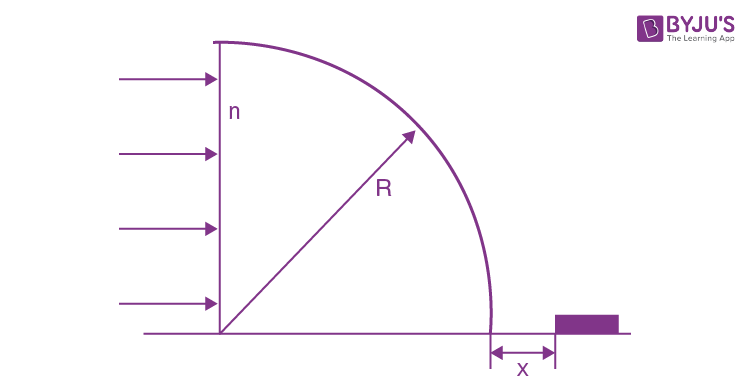1. a. (3√3-4) ×10-2m
2. b. (2√3-2) ×10-2m
3. c. (3√5-5) ×10-2m
4. d. (3√2-3) ×10-2m

Solution: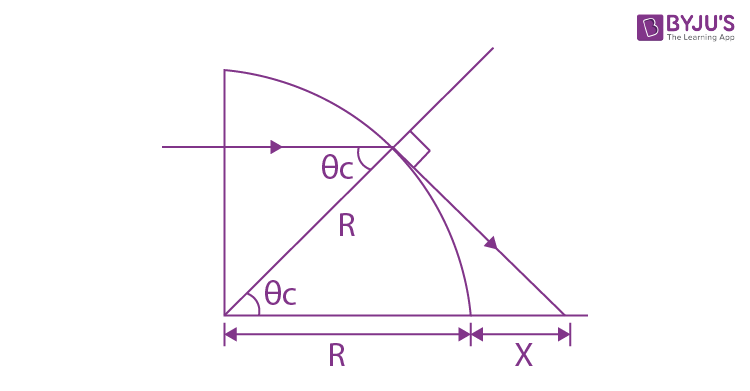sin θc = 1/n = 2/3

cos θc = R/(R+x)

√(1-4/9) = R/(R+x)

√5/3 = R/(R+x)

x = (3√5-5) ×10-2m

Question 22: The de Broglie wavelength of an electron is 0.4×10–10 m when its kinetic energy is 1.0 keV. Its wavelength will be 1.0 ×10–10 m, when its kinetic energy is

1. a. 0.2 keV
2. b. 0.8 keV
3. c. 0.63 keV
4. d. 0.16 keV

Solution:

Wavelength: λ = h/p

= h/√(2mk)

λ ∝1/ √k

√(k2/k1) = λ1/ λ2

(k2/k1) = (λ1/ λ2)2

k2 = (0.4×10-10m/1.0×10-10m)21keV

k2 = 0.16 keV

Question 23: When light of frequency ν1 is incident on a metal with work function W (where h ν1> W), the photocurrent falls to zero at a stopping potential of V1. If the frequency of light is increased to ν2, the stopping potential changes to V2. Therefore, the charge of an electron is given by

1. a. W(ν2+ ν1)/( ν1V2+ ν2V1)
2. b. W(ν2+ ν1)/( ν1V1+ ν2V2)
3. c. W(ν2- ν1)/( ν1V2- ν2V1)
4. d. W(ν21)/( ν2V21V1)

Solution:

We know for a photon:-

KEmax = h ν – h ν0

KEmax = h ν –φ0 .....(i)

KEmax = qV0 .....(ii)

q = charge on electron

V0 = stopping potential

φ0 = work-function of a metal

From (i) & (ii)

qV0 = h ν–φ0

When light of intensity ν1 falls :-

eV1 = h ν1–w

h ν1= w + eV1 ....(iii)

When light of intensity ν2 falls

eV2 = h ν2–w

h ν2 = eV2 + w .....(iv)

(iii) ÷ (iv) :

ν1 / ν2 = (w+eV1)/( w+eV2)

w ν1+ e ν1V2 = w ν2 + e ν2V1

e ν1V2 – e ν2V1 = w[ν2 – ν1]

e = W(ν2- ν1)/( ν1V2- ν2V1)

Question 24: Radon-222 has a half-life of 3.8 days. If one starts with 0.064 kg of Radon-222, the quantity of Radon-222 left after 19 days will be

1. a. 0.002 kg
2. b. 0.062 kg
3. c. 0.032 kg
4. d. 0.024 kg

Solution:

The equation of Radioactive-Decay :-

N=N0e– λt

λ = ln 2/t1/2

= ln 2/3.8

Quantity N at t = 19 day:

N = 0.064 e(-ln2/3.8)19

N = 0.064 e–5ln2

N = 0.064 e–ln32

N = 0.002 kg

Question 25: In the given circuit, the binary inputs at A and B are both 1 in first case and both 0 in the next case. The respective outputs at Y in these two cases will be:

1. a. 1, 1
2. b. 0, 0
3. c. 0, 1
4. d. 1, 0

Solution: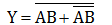For A = 1 ; B = 1

Y = 0

And for A = 0 ; B = 0

Y = 0

Question 26: When a semiconducting device is connected in series with a battery and a resistance, a current is found to flow in the circuit. If, however, the polarity of the battery is reversed, practically no current flows in the circuit. The device may be

1. a. a p-type semiconductor
2. b. a n-type semiconductor
3. c. an intrinsic semiconductor
4. d. a p-n junction

Solution:

p–n junction Diode :

It is a one way device. It offers a low resistance when forward biased hence current easily flow.

It offers high resistance when reverse biased and current almost becomes zero.

Question 27: The dimension of the universal constant of gravitation G is

1. a. [ML2T–1]
2. b. [M–1L3T–2]
3. c. [M–1L2T–2]
4. d. [ML3T–2]

Solution:

From Newton’s law of gravitation:

F = Gm1m2/r2

[G] = [Fr2/m1m2]

= [M1L1T-2 L2/M2]

= [M-1L3T-2]

Question 28: Two particles A and B (both initially at rest) start moving towards each other under a mutual force of attraction. At the instant when the speed of A is v and the speed of B is 2v, the speed of the centre of mass is

1. a. Zero
2. b. v
3. c. 3v/2
4. d. -3v/2

Solution: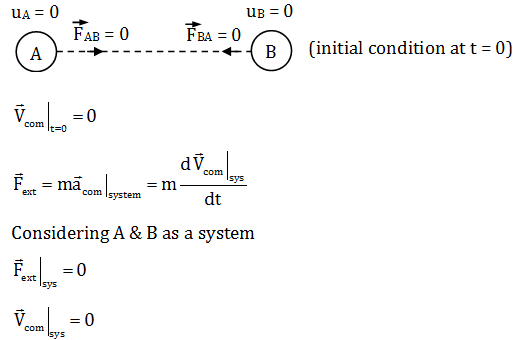Hence, at all instants centre of mass the system will be at rest.

Question 29: Three vectors

$$\vec{A}=a\hat{i}+\hat{j}+\hat{k}$$
,
$$\vec{B}=\hat{i}+b\hat{j}+\hat{k}$$
and
$$\vec{C}=\hat{i}+\hat{j}+c\hat{k}$$
are mutually perpendicular (
$$\hat{i},\hat{j},\hat{k}$$
are unit vectors along X,Yand Z axis respectively). The respective values of a, b and c are

1. a. 0,0,0
2. b. -1/2, -1/2, -1/2
3. c. 1,-1,1
4. d. ½,1/2,1/2

Solution:

There are three unknowns hence we require 3 equations accordingly. [use application of scalar product]

$$\vec{A}.\vec{B}=0$$
[θ = 90°]

a + b + 1 = 0 .....(i)

$$\vec{B}.\vec{C}=0$$

1 + b + c = 0 .....(ii)

$$\vec{C}.\vec{A}=0$$

a + 1 + c = 0 .....(iii)

Adding (i), (ii) & (iii) :

2[a + b + c] + 3 = 0

a = (-3-2(b+c))/2 = -1/2

b = (-3-2(a+c))/2 = -1/2

c = (-3-2(a+b))/2 = -1/2

Question 30: A block of mass 1 kg starts from rest at x = 0 and moves along the x-axis under the action of a force F=kt, where t is time and k =1 Ns–1. The distance the block will travel in 6 seconds is

1. a. 36 m
2. b. 72 m
3. c. 108 m
4. d. 18 m

Solution:

u = 0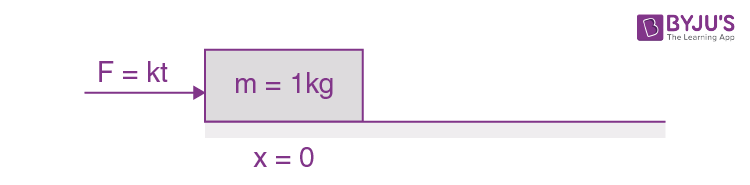F = kt

F = kt = ma

k = 1NS-1

m = 1

t = ma/k = dv/dt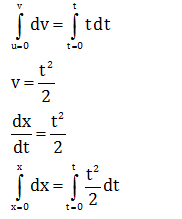x = t3/6

At t = 6 sec, x = 36m.

Question 31: A particle with charge Q coulomb, tied at the end of an inextensible string of length R meter, revolves in a vertical plane. At the centre of the circular trajectory there is a fixed charge of magnitude Q coulomb. The mass of the moving charge M is such that Mg =

$$(\frac{Q^2}{4 \pi \epsilon_0R^2})I$$
. If at the highest position of the particle, the tension of the string just vanishes, the horizontal velocity at the lowest point has to be

1. a. 0
2. b. 2√(gR)
3. c. √(2gR)
4. d. √(5gR)

Solution: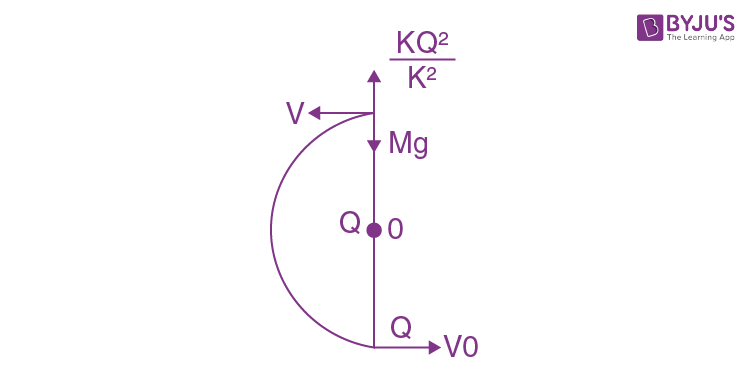As Mg = Q2/4 π ε0R2

At highest point tension in the string vanishes

T = 0

As T = 0

v = 0

Applying work-kinetic-energy theorem:-

WD|All-forces = ∆K.E

Mg[2R] = ½ mv02v0

= 2√(gR)

Question 32: A bullet of mass 4.2×10–2 kg, moving at a speed of 300 ms–1, gets stuck into a block with a mass 9 times that of the bullet. If the block is free to move without any kind of friction, the heat generated in the process will be

1. a. 45 cal
2. b. 405 cal
3. c. 450 cal
4. d. 1701 cal

Solution:

Mass of Bullet = m

Velocity of Bullet = 300 m/s

When Bullet will get stuck inside Block, both will move with same velocity i.e. V

Applying conservation of linear – momentum:-

mv = [m + 9m] V

V = v/10

As there is no friction hence

Heat generated = change in K.E

= ½ mv2-(1/2)10mV2

= ½ mv2-(1/2)10m(v/10)2

= (9/20)mv2

= 1701 J

Total heat generated in calories:-

= 1701/4.2

= 405 cal

Question 33: A particle with charge e and mass m, moving along the X-axis with a uniform speed u, enters a region where a uniform electric field E is acting along the Y-axis. The particle starts to move in a parabola. Its focal length (neglecting any effect of gravity) is

1. a. 2mu2/eE
2. b. eE/2mu2
3. c. mu/2eE
4. d. mu2/2eE

Solution: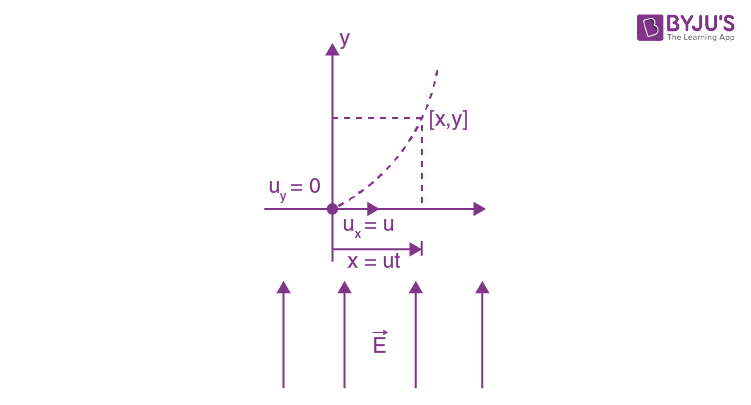ay = Fe/m = [qE/m]

No effect of gravity is considered.

For particle trajectory

Sy = uyt+(1/2)ayt2

y = (1/2)(qE/m)t2

y = (1/2)(qE/m)(x/u)2

y = (Ee/2mu2)x2

Now this parabola relates with

x2 = 4ay

x2 = [2mu2 /Ee] y

4a = 2mu2/Ee

Hence a = mu2/2Ee

Question 34: A unit negative charge with mass M resides at midpoint of the straight line of length 2a adjoining two fixed charges of magnitude + Q each. If it is given a very small displacement x(x < < a) in a direction perpendicular to the straight line, it will

1. a. come back to its original position and stay there
2. b. execute oscillations with frequency 1/2 π √(Q/4πε0Ma3)
3. c. fly to infinity
4. d. execute oscillations with frequency 1/2 π √(Q/4πε0Ma2)

Solution:

BonusIts given small displacement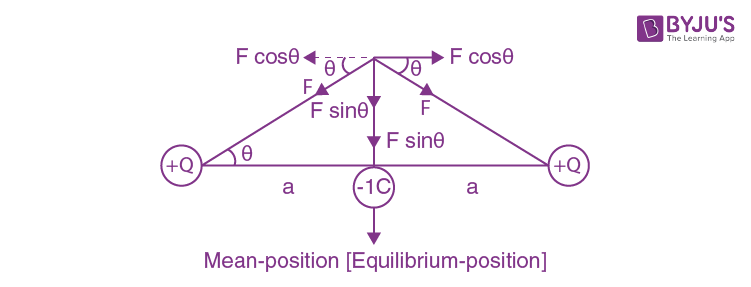Restoring force towards mean position

Fnet = -2Fsin θ

Fnet = [-2KQ×1/(y2+a2)](y/√(y2+a2)

= -2KQy/[y2+a2]3/2

y<<a small displacement

Fnet = -2KQy/a3

Frequency [f] = (1/2π)√(K/M)

= (1/2π)√(2KQ/Ma3)

= (1/2π)√(Q/2π ε0Ma3)

None of the option is correct.

Question 35: Consider the circuit given here. The potential difference VBC between the points B and C is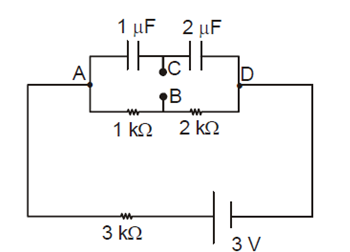1. a. 1V
2. b. 0.5 V
3. c. 0v
4. d. –1V

Solution:

At starting of the circuit [t = 0] capacitor can be replaced by a simple wire. Hence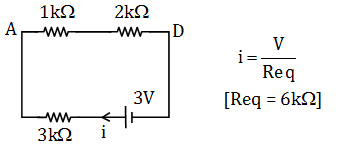i = 3/6×103

= 0.5×10–3 A

= 0.5 × 10–3×3×103

= 1.5 V

Q = (2/3)1.5 = 1μC

Applying KVL from B to C

VB – 0.5×10–3 ×2×103 +(1/2) = VC

VB–VC = 0.5 V

Question 36: If the pressure, temperature and density of an ideal gas are denoted by P, T and ρ, respectively, the velocity of sound in the gas is

1. a. Proportional to √P , when T is constant.
2. b. Proportional to √T
3. c. Proportional to √P , when ρ is constant.
4. d. Proportional to T.

Solution:

Velocity of sound: V = √(γRT/M)….(i)

PV = nRT

PV = (m/M)RT

P = (m/V)RT/M

P = ρRT/M

RT/M = P/ρ

Putting value in (i)

V = √(γP/ρ) …(ii)

Now from (i) and (ii)

V ∝ √T

When ρ is constant

V ∝ √P

Option (b) and (c) are correct.

Question 37: Two long parallel wires separated by 0.1 m carry currents of 1 A and 2 A respectively in opposite directions. A third current-carrying wire parallel to both of them is placed in the same plane such that it feels no net magnetic force. It is placed at a distance of

1. a. 0.5 m from the 1st wire, towards the 2nd wire.
2. b. 0.2 m from the 1st wire, towards the 2nd wire.
3. c. 0.1 m from the 1st wire, towards the 2nd wire.
4. d. 0.2 m from the 1st wire, away from the 2nd wire.

Solution: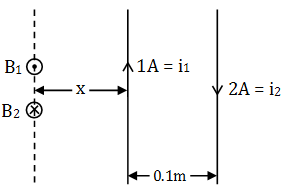B1 = B2

μ0(1)/2 πx = μ0(2)/2 π(0.1+x)

x = 0.1 m

Question 38: If χ stands for the magnetic susceptibility of a substance, µ for its magnetic permeability and µ0 for the permeability of free space, then

1. a. for a paramagnetic substance : χ > 0, µ > 0
2. b. for a paramagnetic substance : χ > 0, µ > µ0
3. c. for a diamagnetic substance : χ < 0, µ < 0
4. d. for a ferromagnetic substance : χ > 1, µ < µ0

Solution:

χ = μr–1

μr = μ/μ0

For paramagnetic substance:- χ > 0, μr > 1 ; μ > μ0

For diamagnetic substance: χ < 0, μr < 1 ; μ < μ0

For ferromagnetic substance:- χ >> 1, μ >> μ0

If 0 <μ <μ0 then substance will not be paramagnetic. Hence option (a) is incorrect.

Question 39: Let vn and En be the respective speed and energy of an electron in the nth orbit of radius rn, in a hydrogen atom, as predicted by Bohr’s model. Then

1. a. plot of Enrn/E1r1 as a function of n is a straight line of slope 0.
2. b. plot of rnvn/r1v1 as a function of n is a straight line of slope 1.
3. c. plot of ln (rn/rn) as a function of ln(n) is a straight line of slope 2.
4. d. plot of ln (rn E1/Enrn) as a function of ln(n) is a straight line of slope 4.

Solution: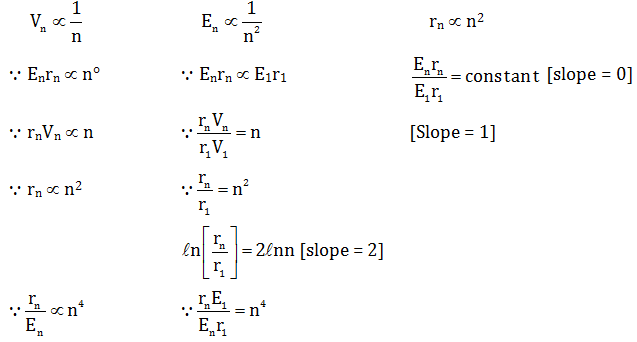ln(rnE1/r1En) = 4ln n (slope = 4)

Option a,b,c,d are correct.

Question 40: A small steel ball bounces on a steel plate held horizontally. On each bounce the speed of the ball arriving at the plate is reduced by a factor e (coefficient of restitution) in the rebound, so that Vupward = eVdownward

If the ball is initially dropped from a height of 0.4 m above the plate and if 10 seconds later the bouncing ceases, the value of e is

1. a. √(2/7)
2. b. 3/4
3. c. 13/18
4. d. 17/18

Solution:

Total time of bouncing = 10 sec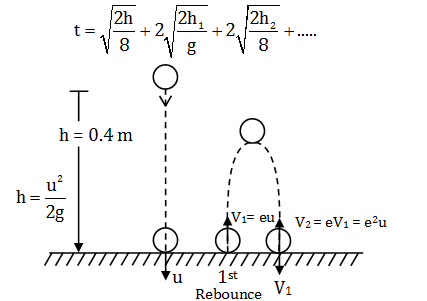Maximum height achieved in 1st rebounce :

h1 = V12/2g = e2u2/2g = he2

Time taken in 1st rebounce :

t1 = 2 √(2h1/g)

= 2√(he2/g)

Time taken in 2nd rebounce :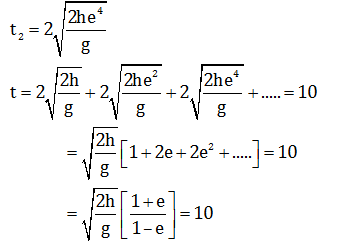e = 17/18

### WBJEE 2017 Physics Question Paper with Solutions